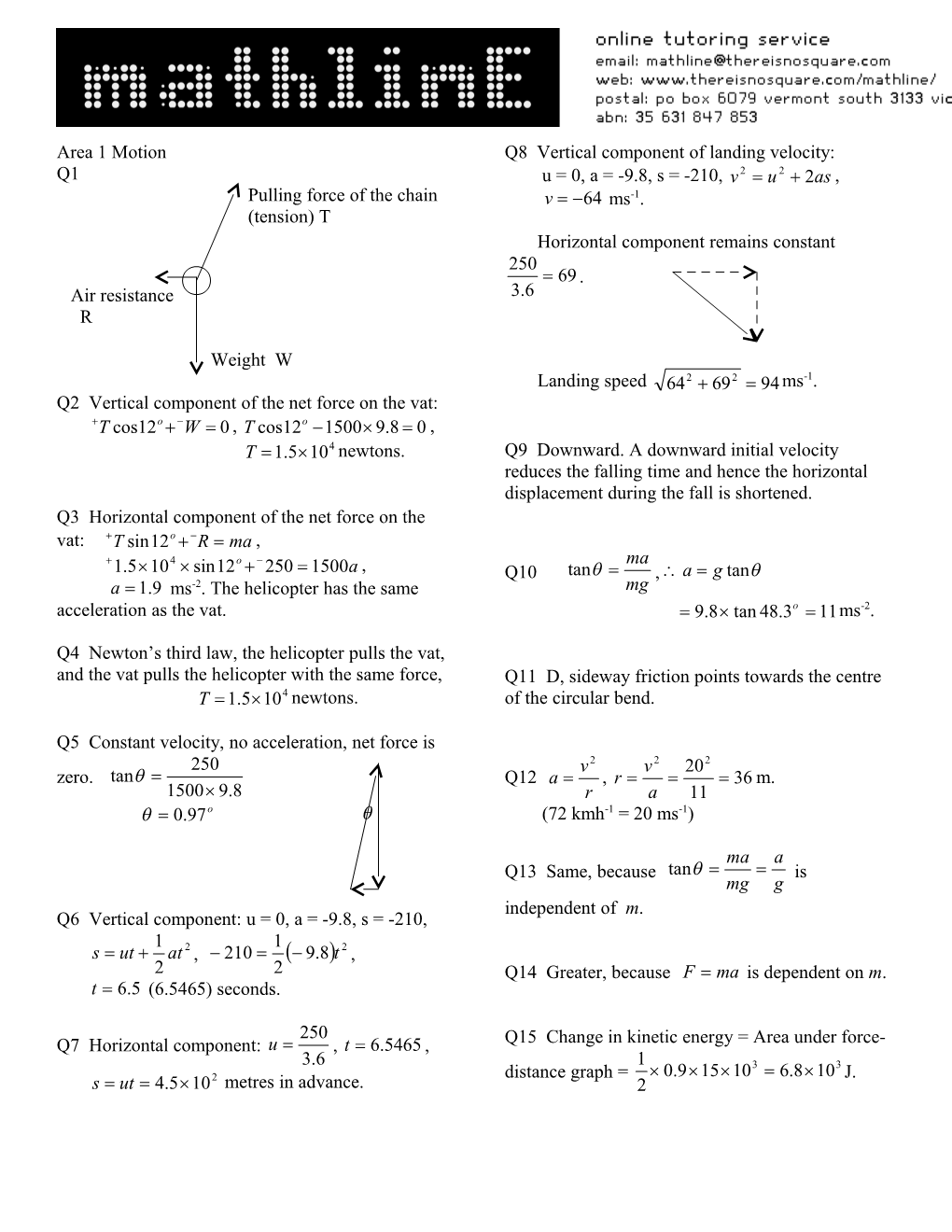# Pulling Force of the ChainArea 1 Motion

Q1

Pulling force of the chain

(tension) T

Air resistance

R

Weight W

Q2 Vertical component of the net force on the vat:

, ,

newtons.

Q3 Horizontal component of the net force on the vat: ,

,

ms-2. The helicopter has the same acceleration as the vat.

Q4 Newton’s third law, the helicopter pulls the vat, and the vat pulls the helicopter with the same force,

newtons.

Q5 Constant velocity, no acceleration, net force is zero.

Q6 Vertical component: u = 0, a = -9.8, s = -210,

, ,

(6.5465) seconds.

Q7 Horizontal component: , ,

Q8 Vertical component of landing velocity:

u = 0, a = -9.8, s = -210, ,

ms-1.

Horizontal component remains constant

.

Landing speed ms-1.

Q9 Downward. A downward initial velocity reduces the falling time and hence the horizontal displacement during the fall is shortened.

Q10 ,

ms-2.

Q11 D, sideway friction points towards the centre of the circular bend.

Q12 , m.

(72 kmh-1 = 20 ms-1)

Q13 Same, because is independent of m.

Q14 Greater, because is dependent on m.

Q15 Change in kinetic energy = Area under force-distance graph = J.

Q16 ,

,

ms-1.

The speed of the car and the speed of the dummy are the same.

Q17 The seatbelt keeps the occupant moving together with the car while the car slows down abruptly in a crash. The crumple zone lengthens the stopping distance of the car and hence lengthens the stopping distance of the occupant.

Since where F is the force of the seatbelt on the occupant and d the stopping distance, for the same , . Therefore larger d results in smaller F on the occupant.

Q18 Total momentum is conserved.

,

kmh-1 east.

Q19 Just before collision, total EK = joules

Just after collision, total EK =

joules.

There is a loss of kinetic energy, collision is inelastic.

Q20 Impulse = change in momentum

= final momentum – initial momentum

=

= i.e. 1.9M Ns west.

Area 2 Gravity

Q1

ms-2

Q2 Weight

newtons.

Q3 The astronaut was in free fall, i.e. he moved under the influence of gravity only and there was no reaction force on him. Zero reaction force resulted in zero apparent weight (weight experienced by the astronaut), therefore the astronaut felt weightless.

Q4 Zero, because the astronaut, the spring balance and the mass would all be in free fall. They would not exert force on each other.

Q5 Both would move in the same orbit as that before they were released. They would move along the side of the astronaut unless they were pushed.

Q6 Since , ,

.

earth year, ,

earth years.

Q7 Since ,

Area 3 Structures and materials

Q1 A structure is in stable equilibrium if it returns to its original position after it is given a small angular displacement. This is possible when it has a relatively wide base, and the line of its weight force passes through the base.

Q2 The tower is on the verge of toppling over when the line of its weight force passes through the edge of its base. 7m

55m

Q3 Since the top is displaced 4.6 m from the vertical, therefore the centre of mass is displaced 2.3 m from the vertical, and the line of the weight force is 2.3 m from O.

Torque of the weight force about O =

Nm

Minimum torque of the pull = 23 Nm

Q4 Pa

Q5 D

Q6 C

Q7 Concrete can be strengthened by placing steel rods in tension while the concrete is poured and allowed to dry before the tension is released. The contraction of the rods after the release of the tension keeps the concrete in compression all the time so that existing cracks do not propagate. It will be in compression provided the load on it is below the recommended limit.

Q8 A stiffer material has a higher Young’s modulus.

For A, Young’s modulus = MPa

= 2500000 MPa

= 2500 GPa

For B, Young’s modulus = MPa

= 2000000 MPa

= 2000 GPa

A is stiffer than B. Both are very stiff.

Q9 A tougher material absorbs more strain energy per cubic metre (area under graph) before it fractures.

For A, area under graph

For B, area under graph

B is tougher than A.

Q10 Strength is the maximum stress a material can withstand before it fractures.

Strength of A is 250 MPa (MNm-2).

Q11 ,

Area 4 Ideas about light and matter

Q1 D. A material has its own threshold frequency for the emission of photoelectrons. The chosen frequency must be higher than the threshold frequency for it to occur.

Q2 D. Higher intensity produces more photoelectrons and hence higher current.

Q3 C. Higher frequency produces photoelectrons with higher kinetic energy and hence higher retarding voltage is required to stop all electrons from reaching the opposite plate.

Q4 According to the wave model, light is a continuous wave and its energy is related to the amplitude of the wave. When electrons in a metal is exposed to light, the amount of energy absorbed by each electron depends on the time of exposure and therefore can be any value. This model fails to explain that (a) more photoelectrons with light of higher intensity and (b) higher kinetic energy of photoelectrons with light of higher frequency. According to the photon model, a higher intensity light beam has more photons, and the energy of each photon is directly proportional to the frequency of the light beam. Greater number of photons in the beam provides the electrons with a higher probability to absorb a photon and thus more photoelectrons are emitted. Also higher frequency photons give the electrons more kinetic energy and thus a higher retarding voltage is required.

Q5 Photon energy , ,

.

Q6 Energy from UV light bulb in a minute

Energy of a UV photon

Number of photons

.

Q7

Q8 de Broglie wavelength

m.